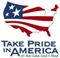Scientific Investigations Report 2009–5014

U.S. GEOLOGICAL SURVEY
Scientific Investigations Report 2009–5014

## Conversion Factors and Datums

### Conversion Factors

Multiply By To obtain
Length
inch (in.) 2.54 centimeter (cm)
foot (ft) 0.3048 meter (m)
foot per mile (ft/mi) 0.1894 meter per kilometer (m/km)
mile (mi) 1.609 kilometer (km)
Area
acre 4,047 square meter (m2)
square foot per day (ft2/d) 0.09290 square meter per day (m2/d)
square foot (ft2) 0.09290 square meter (m2)
square mile (mi2) 2.590 square kilometer (km2)
Volume
acre-foot (acre-ft) 1,233 cubic meter (m3)
Flow rate
cubic foot per second (ft3/s) 0.02832 cubic meter per second (m3/s)

Transmissivity: The standard unit for transmissivity is cubic foot per day per square foot times foot of aquifer thickness [(ft3/d)/ft2]ft. In this report, the mathematically reduced form, foot squared per day (ft2/d), is used for convenience.

### Datums

Vertical coordinate information is referenced to the National Geodetic Vertical Datum of 1929 (NGVD 29).

Horizontal coordinate information is referenced to the North American Datum of 1927 (NAD 27).

Altitude, as used in this report, refers to distance above the vertical datum.U.S. Department of the Interior | U.S. Geological Survey